# Setup for 4 Player a Game of Food Chain Magnate

Originally generated on 1/11/2019 7:22:26 AM
A direct link to this setup is http://www.VentersConsulting.com/BoardgameHelpers/FoodChainMagnateGenerator.aspx?LoadGame=\$2a\$04\$qo1tgTsQRAvTm2y11JHPQu

## Player Setup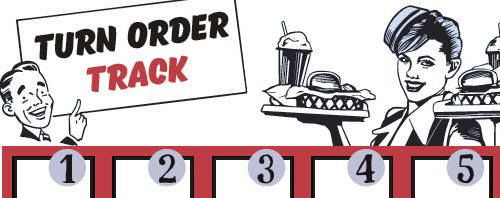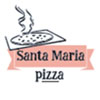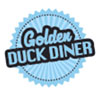## Other Setup

Bank starts with \$200.00
Remove these Billboards: #16
Number of 1x Employee cards used = 2

## Map (Random)

Click here to view Map Tile Lettering Key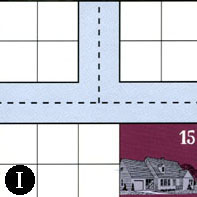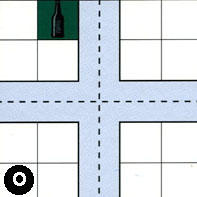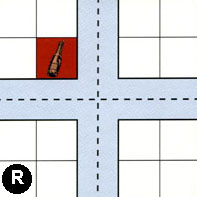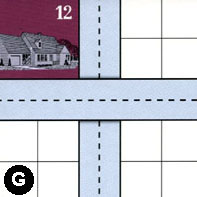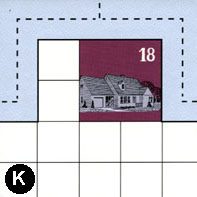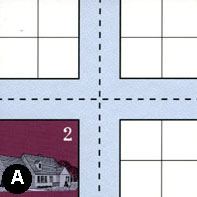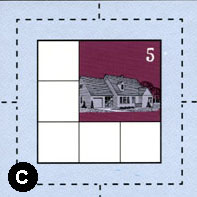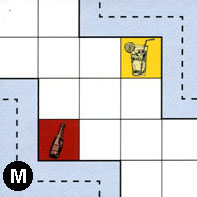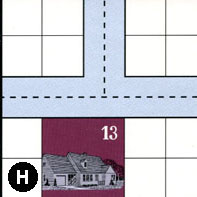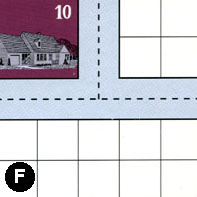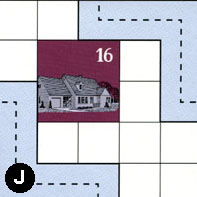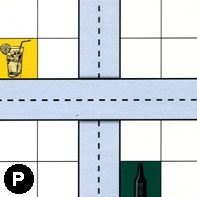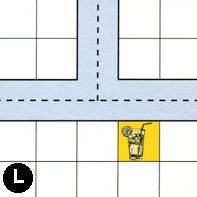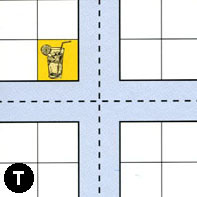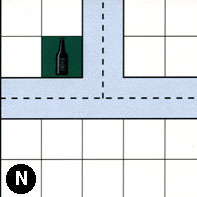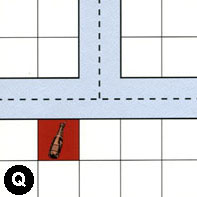### Map Stats

Map Option = Random
Total Number of Tiles = 16
Total Number of Starting Houses = 8
Total Number of Beer Spots = 3
Total Number of Soda Spots = 3
Total Number of Lemonade Spots = 4
Total Number of Drink Spots = 10
Number of independent path systems = 2
Number of independent neighborhoods = 17

Path System 1
Size = Medium
Number of Tiles = 15
Number of Paths = 54
Contains Loop(s) = No
Number of Starting Houses = 9
Starting Houses Pct of Map (%) = 112
Number of Beer Spots = 2
Number of Soda Spots = 2
Number of Lemonade Spots = 3
Number of Drink Spots = 7
Drink Spots Pct of Map (%) = 70
Tile Ids = A, C, F, G, H, I, J, K, L, M, N, O, P, R, T
Paths = IWN, OWN, AWN, KWN, HWN, FWN, TWN, NWN, NWE, JWS, PWE, NNE, TNE, TWE, FWE, AWS, KWE, KNE, HWE, LWE, LWN, LNE, HNE, FNE, ANS, OWE, ONE, INE, IWE, ONS, RWN, CWN, MWS, GNS, CNE, JEN, CWE, RWE, RNE, OWS, ANE, CWS, RWS, GWE, RNS, OES, RES, CNS, CES, AWE, AES, TWS, TNS, TES What do these mean?
Starting House Ids = 0, 2, 5, 10, 12, 13, 15, 16, 18
Path System 2
Size = Medium
Number of Tiles = 3
Number of Paths = 5
Contains Loop(s) = No
Number of Starting Houses = 1
Starting Houses Pct of Map (%) = 12
Number of Beer Spots = 1
Number of Soda Spots = 1
Number of Lemonade Spots = 1
Number of Drink Spots = 3
Drink Spots Pct of Map (%) = 30
Tile Ids = M, P, Q
Paths = MEN, PNS, QWN, QWE, QNE What do these mean?
Starting House Ids = 0

Neighborhood 1
Total Size = Large
Number of Total Spaces = 38
Number of Empty Spaces (for new houses & gardens) = 29
Number of Beer Spots = 1
Number of Soda Spots = 0
Number of Lemonade Spots = 0
Number of Drink Spots = 1
Number of Starting Houses = 2
Starting Houses Pct of Map (%) = 25
Tile Ids = A, I, K, O
Starting House Ids = 15, 18
Neighborhood 2
Total Size = Medium
Number of Total Spaces = 8
Number of Empty Spaces (for new houses & gardens) = 8
Number of Beer Spots = 0
Number of Soda Spots = 0
Number of Lemonade Spots = 0
Number of Drink Spots = 0
Number of Starting Houses = 0
Starting Houses Pct of Map (%) = 0
Tile Ids = I, O
Neighborhood 3
Total Size = Medium
Number of Total Spaces = 8
Number of Empty Spaces (for new houses & gardens) = 8
Number of Beer Spots = 0
Number of Soda Spots = 0
Number of Lemonade Spots = 0
Number of Drink Spots = 0
Number of Starting Houses = 0
Starting Houses Pct of Map (%) = 0
Tile Ids = O, R
Neighborhood 4
Total Size = Medium
Number of Total Spaces = 8
Number of Empty Spaces (for new houses & gardens) = 4
Number of Beer Spots = 0
Number of Soda Spots = 0
Number of Lemonade Spots = 0
Number of Drink Spots = 0
Number of Starting Houses = 1
Starting Houses Pct of Map (%) = 12
Tile Ids = G, R
Starting House Ids = 12
Neighborhood 5
Total Size = Small
Number of Total Spaces = 4
Number of Empty Spaces (for new houses & gardens) = 4
Number of Beer Spots = 0
Number of Soda Spots = 0
Number of Lemonade Spots = 0
Number of Drink Spots = 0
Number of Starting Houses = 0
Starting Houses Pct of Map (%) = 0
Tile Ids = G
Neighborhood 6
Total Size = Medium
Number of Total Spaces = 12
Number of Empty Spaces (for new houses & gardens) = 11
Number of Beer Spots = 0
Number of Soda Spots = 1
Number of Lemonade Spots = 0
Number of Drink Spots = 1
Number of Starting Houses = 0
Starting Houses Pct of Map (%) = 0
Tile Ids = A, O, R
Neighborhood 7
Total Size = Medium
Number of Total Spaces = 8
Number of Empty Spaces (for new houses & gardens) = 8
Number of Beer Spots = 0
Number of Soda Spots = 0
Number of Lemonade Spots = 0
Number of Drink Spots = 0
Number of Starting Houses = 0
Starting Houses Pct of Map (%) = 0
Tile Ids = G, R
Neighborhood 8
Total Size = Large
Number of Total Spaces = 60
Number of Empty Spaces (for new houses & gardens) = 52
Number of Beer Spots = 1
Number of Soda Spots = 1
Number of Lemonade Spots = 2
Number of Drink Spots = 4
Number of Starting Houses = 1
Starting Houses Pct of Map (%) = 12
Tile Ids = A, F, G, J, M, N, P, T
Starting House Ids = 16
Neighborhood 9
Total Size = Medium
Number of Total Spaces = 9
Number of Empty Spaces (for new houses & gardens) = 5
Number of Beer Spots = 0
Number of Soda Spots = 0
Number of Lemonade Spots = 0
Number of Drink Spots = 0
Number of Starting Houses = 1
Starting Houses Pct of Map (%) = 12
Tile Ids = C
Starting House Ids = 5
Neighborhood 10
Total Size = Medium
Number of Total Spaces = 12
Number of Empty Spaces (for new houses & gardens) = 8
Number of Beer Spots = 0
Number of Soda Spots = 0
Number of Lemonade Spots = 0
Number of Drink Spots = 0
Number of Starting Houses = 1
Starting Houses Pct of Map (%) = 12
Tile Ids = A, F, H
Starting House Ids = 2
Neighborhood 11
Total Size = Medium
Number of Total Spaces = 14
Number of Empty Spaces (for new houses & gardens) = 10
Number of Beer Spots = 0
Number of Soda Spots = 0
Number of Lemonade Spots = 0
Number of Drink Spots = 0
Number of Starting Houses = 1
Starting Houses Pct of Map (%) = 12
Tile Ids = H, L
Starting House Ids = 13
Neighborhood 12
Total Size = Small
Number of Total Spaces = 4
Number of Empty Spaces (for new houses & gardens) = 4
Number of Beer Spots = 0
Number of Soda Spots = 0
Number of Lemonade Spots = 0
Number of Drink Spots = 0
Number of Starting Houses = 0
Starting Houses Pct of Map (%) = 0
Tile Ids = P
Neighborhood 13
Total Size = Medium
Number of Total Spaces = 26
Number of Empty Spaces (for new houses & gardens) = 21
Number of Beer Spots = 0
Number of Soda Spots = 0
Number of Lemonade Spots = 1
Number of Drink Spots = 1
Number of Starting Houses = 1
Starting Houses Pct of Map (%) = 12
Tile Ids = F, H, L, T
Starting House Ids = 10
Neighborhood 14
Total Size = Large
Number of Total Spaces = 28
Number of Empty Spaces (for new houses & gardens) = 27
Number of Beer Spots = 0
Number of Soda Spots = 1
Number of Lemonade Spots = 0
Number of Drink Spots = 1
Number of Starting Houses = 0
Starting Houses Pct of Map (%) = 0
Tile Ids = N, P, Q
Neighborhood 15
Total Size = Medium
Number of Total Spaces = 8
Number of Empty Spaces (for new houses & gardens) = 7
Number of Beer Spots = 0
Number of Soda Spots = 0
Number of Lemonade Spots = 1
Number of Drink Spots = 1
Number of Starting Houses = 0
Starting Houses Pct of Map (%) = 0
Tile Ids = P, Q
Neighborhood 16
Total Size = Small
Number of Total Spaces = 4
Number of Empty Spaces (for new houses & gardens) = 4
Number of Beer Spots = 0
Number of Soda Spots = 0
Number of Lemonade Spots = 0
Number of Drink Spots = 0
Number of Starting Houses = 0
Starting Houses Pct of Map (%) = 0
Tile Ids = L
Neighborhood 17
Total Size = Medium
Number of Total Spaces = 8
Number of Empty Spaces (for new houses & gardens) = 7
Number of Beer Spots = 1
Number of Soda Spots = 0
Number of Lemonade Spots = 0
Number of Drink Spots = 1
Number of Starting Houses = 0
Starting Houses Pct of Map (%) = 0
Tile Ids = N, T

v8.3.2